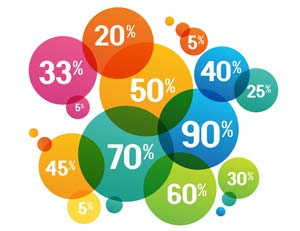# Percentage Calculators

of
is ? % of
to:
is:of?
Use these percentage calculator tools to work out increases, decreases or percentage differences. Common uses include calculating tax, statistics, savings increases, or tips on a restaurant bill. Formulae and explanations appear further down the page.

Disclaimer: Whilst every effort has been made in building our calculator tools, we are not to be held liable for any damages or monetary losses arising out of or in connection with their use. Full disclaimer.

## What is X percent of Y?

In order to explain this calculation to you as easily as possible, we'll replace the X and Y with a couple of figures. Let's say the question is: What is 10% of 200?

1. Our first step is to change the percentage figure into a decimal. We do this by dividing the figure by 100. So, 10% becomes 10/100 = 0.1.
2. The next step is to multiply the decimal figure by the full amount. So, 0.1 x 200 = 20.
4. In equation form, where Z is your answer, your equation is Z = (X/100) * Y.

## X is what percent of Y?Once again, we'll replace the X and Y with a couple of figures to explain this. Let's say the question is: 15 is what percent of 300?

1. Our first step is to divide the X figure by the Y figure. So, in the case of our example: 15/300 = 0.05.
2. Step 1 has given you a decimal figure. To convert this into a percentage figure we need to multiply by 100. So, 0.05 x 100 = 5.
4. In equation form, where Z is your answer, your equation is Z = (X/Y) * 100.

## What is the percentage increase or decrease?

Continuing the theme, let's replace the X and Y with a couple of figures. Let's say the question is: What is the percentage increase from 40 to 68? We can use the percentage change calculator. Or we can work the calculation out manually.

1. Our first step is to subtract X from Y and then divide it by X. In the case of our example it would be (68-40) / 40. Note that we've put brackets around the first part to ensure this is done first (see our article on PEMDAS and the order of operations for explanation).
2. (68-40) / 40 gives us a result of 0.7. As this is a decimal, we convert it into a percentage figure by multiplying it by 100. So, 0.7 x 100 = 70.
3. This gives you your answer. The percentage increase from 40 to 68 = 70%.
4. In equation form, where Z is your answer, your equation is Z = (Y-X) / X * 100.

## X is Y percentage of what number?

Let's replace the X and Y with a couple of figures. Let's say the question is: 5 is 20% of what number?

1. Step one is to change the Y percentage figure into a decimal. We do this by dividing the figure by 100. So, 20% becomes 20/100 = 0.2.
2. Next, we divide our X figure by our Y figure. so, 5 / 0.2 = 25.